# Data Forecasting and Segmentation Using Microsoft Excel5 (1 reviews total)
By Fernando Roque
• \$35.99 eBook
• \$44.99 Print + eBook
• \$12.99 eBook + Subscription
What do you get with a Packt Subscription?

• Constantly updated with 100+ new titles each month
• Breadth and depth in over 1,000+ technologies

Data Forecasting and Segmentation Using Microsoft Excel guides you through basic statistics to test whether your data can be used to perform regression predictions and time series forecasts. The exercises covered in this book use real-life data from Kaggle, such as demand for seasonal air tickets and credit card fraud detection.

You’ll learn how to apply the grouping K-means algorithm, which helps you find segments of your data that are impossible to see with other analyses, such as business intelligence (BI) and pivot analysis. By analyzing groups returned by K-means, you’ll be able to detect outliers that could indicate possible fraud or a bad function in network packets.

By the end of this Microsoft Excel book, you’ll be able to use the classification algorithm to group data with different variables. You’ll also be able to train linear and time series models to perform predictions and forecasts based on past data.

Publication date:
May 2022
Publisher
Packt
Pages
324
ISBN
9781803247731

# Chapter 1: Understanding Data Segmentation

Machine learning has two types of algorithms depending on the level of adjustments that you require to give a response:

• Supervised
• Unsupervised

Supervised algorithms need continuous improvement in the form of the data used to train them. For example, a supervised machine learning function of a linear model needs a starter group of data to train and generate the initial conditions. Then, we have to test the model and use it. We need continuous surveillance of the results to interpret whether they make sense or not. If the model fails, we probably need to train the model again.

Unsupervised algorithms do not require any previous knowledge of the data. The unsupervised machine learning process takes data and starts analyzing it until it reaches a result. Contrary to supervised linear regression and time series, this data does not need a test to see whether it is useful to build a model. That is the case with the K-means algorithm, which takes unknown and untested data to classify the values of the variables and returns the classification segments.

In this book, we will cover three different topics of machine learning:

• Grouping statistics to find data segments
• Linear regression
• Time series

For grouping statistics, we will use an add-on for Excel that will do the classification automatically for us. This add-on is included with the book, and we will learn how to use it throughout this book. For linear regression, we will use Excel formulas to find out whetherthe data can be used to make predictions with regression models and forecasts from the time series.

We need a machine learning algorithm to classify and group data for the following reasons:

• A large amount of data is difficult to classify manually.
• Segmentation by observing a 2D or 3D chart is not accurate.
• Segmenting multiple variables is impossible because it is not possible to do a chart of multiple dimensions.

Before we do group segmentation using K-means clustering, we need to find the optimal number of groups for our data. The reason for this is that we want compact groups with points close to the average value of the group. It is not a good practice to have scattered points that do not belong to any group and that could be outliers that do not perform like the rest of the data, as they could be anomalies that deserve further research.

The K-means function will also help to get the optimal number of groups for our data. The best-case scenario is to have compact groups with points near their center.

We will review the basic statistical concepts to work with data grouping. These concepts are as follows:

• Mean
• Standard deviation

In the data grouping segment, the mean is the center, or centroid, of the group. The best case is that the values are compact and close to the segment's centroid.

The level of separation of the values within a group from its centroid is measured by the standard deviation. The best case is to have compact groups with values close to the group's mean point with a low standard deviation for each group.

When we have values and segments that are scattered with a large standard deviation, that means they are outliers. Outliers are data that behaves differently from the majority of other segments. It is a special kind of data because it requires further research. Outliers could indicate an anomaly that could grow and cause a problem in the future. Practical examples of outliers that require attention are as follows:

• Values that are different from the normal transaction amounts in sales and purchases. These could indicate a system test that could lead to a bigger issue in the future.
• A timeline of suspicious system performance. This could indicate hacking attempts.

In this chapter, we will cover the following topics:

• Segmenting data concepts
• Grouping data in segments of two and three variables

# Segmenting data concepts

Before explaining data segments, we have to review basic statistical concepts such as mean and standard deviation. The reason is that each segment has a mean, or central, value, and each point is separated from the central point. The best case is that this separation of points from the mean point is as small as possible for each segment of data.

For the group of data in Figure 1.1, we will explain the mean and the separation of each point from the center measured by the standard deviation: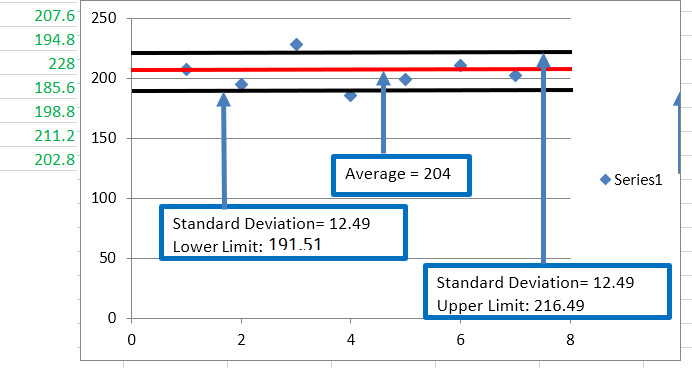Figure 1.1 – Average, standard deviation, and limits. The data on the left is represented in the chart

The mean of the data on the left of the chart is 204. The group's centroid is represented by the middle line in Figure 1.1.

The standard deviation for this data is 12.49. So, the data upper limit is 216.49 and the lower limit is 191.51.

The standard deviation is the average separation of all the points from the centroid of the segment. It affects the grouping segments, as we want compact groups with a small separation between the group's data points. A small standard deviation means a smaller distance from the group's points to the centroid. The best case for the data segments is that these data points are as close as possible to the centroid. So, the standard deviation of the segment must be a small value.

Now, we will explore four segments of a group of data. We will find out whether all the segments are optimal, and whether the points are close to their respective centroids.

In Figure 1.2, the left column is sales revenue data. The right column is the data segments: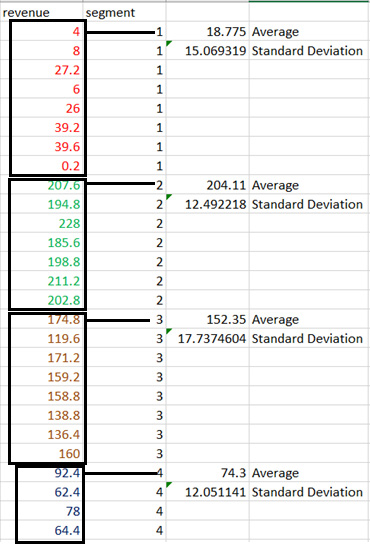Figure 1.2 – Segments, mean, and standard deviation

We have four segments, and we will analyze the mean and the standard deviation to see whether the points have an optimal separation from the centroid. The separation is given by the standard deviation.

Figure 1.3 is the chart for all the data points in Figure 1.2. We can identify four possible segments by simple visual analysis: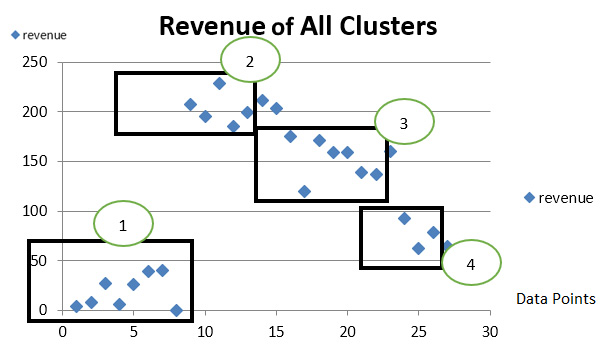Figure 1.3 – Data segments

We will analyze the centroid and the separation of the points for each segment in Figure 1.3. We can see that the group between 0 and 60 on the y axis is probably an outlier because the revenue is very low compared with the rest of the segments. The other groups appear to be compact around their respective centroid. We will confirm this in the charts of each segment.

The mean for the first segment is 18.775. The standard deviation is 15.09. That means there is a lot of variation around the centroid. This segment is not very compact, as we can see in Figure 1.4. The data is scattered and not close to the centroid value of 18.775: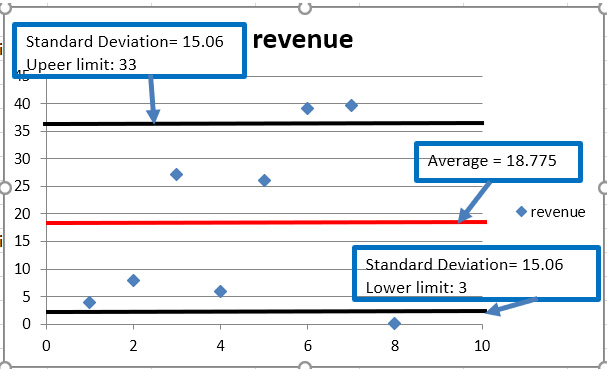Figure 1.4 – Segment 1, mean and standard deviation

The centroid of this segment is 18.775. The separation of the points measured by the standard deviation is 15.06. The points fall in the range of 3 to 33. That means the separation is wide and the segment is not compact. An explanation for this type of segment is that the points are outliers. They are points that do not have normal behavior and deserve special analysis to research. When we have points that are outside the normal operation values, for example, transactions with smaller amounts than normal at places and times that do not correspond to the rest of the data, we have to do deeper research because they could be indicators of fraud. Or, maybe they are sales that occur only at specific times of the month or year.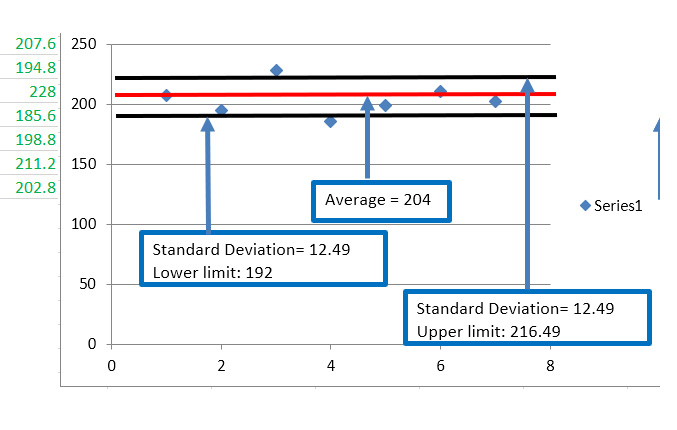Figure 1.5 – Segment 2, mean and standard deviation

The second segment is more compact than the first one. The mean is 204 and there's a small standard deviation of 12.49. The upper limit is 216 and the lower limit is 192. This is an example of a good segmentation group. The distance from the data points to the centroid is small.

Next is segment number three: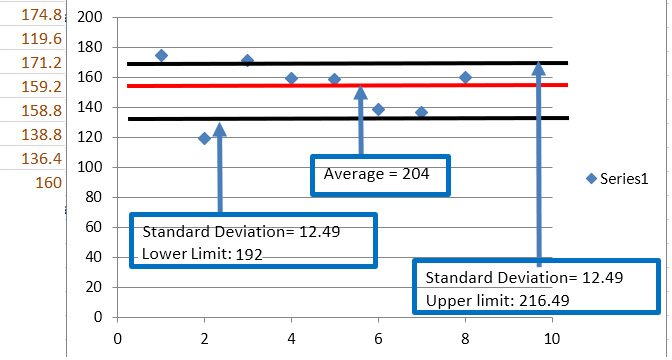Figure 1.6 – Segment 3, mean and standard deviation

The mean is 204, the upper limit is 216, and the lower limit is 192. By the standard deviation of the points, we also conclude that the segment is compact enough to give reliable information.

The points are close to the centroid, so the behavior of the members of the group or segment is very similar.

Segment number four is the smallest of all. It is shown in Figure 1.7: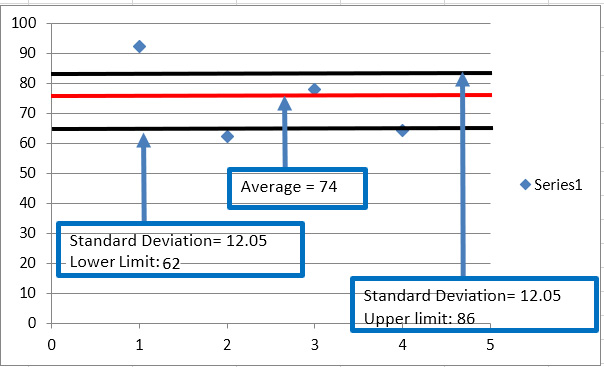Figure 1.7 – Segment 3, mean and standard deviation

The limits are 62 and 86 and the mean is 74. Figure 1.3 shows that segment four is the group with the second-lowest revenue after segment one. But segment one is scattered with a large standard deviation, so it is a not compact group, and the information is not reliable.

After reviewing the four segments, we conclude that segment number one is the lowest revenue group. It also has the highest separation of points from its centroid. It is probably an outlier and represents the non-regular behavior of sales.

In this section, we reviewed the basic statistical concepts and how they relate to segmentation. We learned that the best-case scenario is to have compact groups with a small standard deviation from the group's mean. It is important to follow up on the points that are outside the groups. These outliers (with very different behavior compared with the rest of the values) could be indicators of fraud. In the next section, we will apply these concepts to multi-variable analysis. We will have groups with two or more variables.

# Grouping data in segments of two and three variables

Now, we are going to segment data with two variables. Several real-world problems need to group two or more variables to classify data where one variable influences the other. For example, we can use the month number and the sales revenue dataset to find out the time of the year with higher and lower sales. We will use online marketing and sales revenue. Figure 1.8 shows the four segments of the data and the relationship between online marketing investment and revenue. We can see that segments 1, 2, and 4 are relatively compact. The exception is segment 3 because it has a point that appears to be an outlier. This outlier will affect the average and the standard deviation of the segment: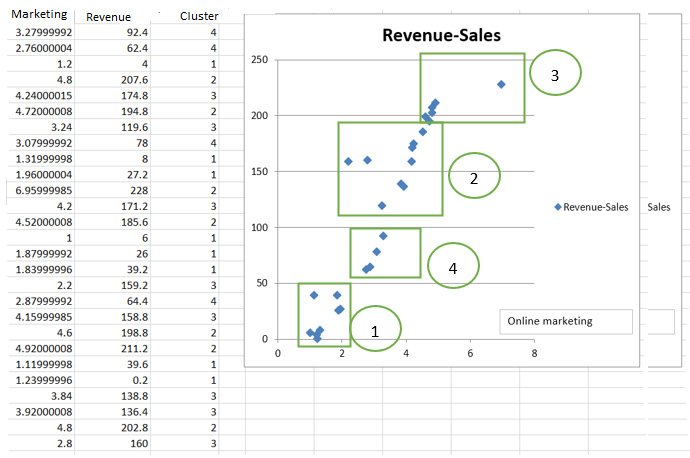Figure 1.8 – Grouping with two variables

Segment 4 appears to have the smallest standard deviation. This group looks compact. Segment 2 also appears to be compact and it has a high value of revenue.

In Figure 1.9, we will find out the mean and the standard deviation of segment 2: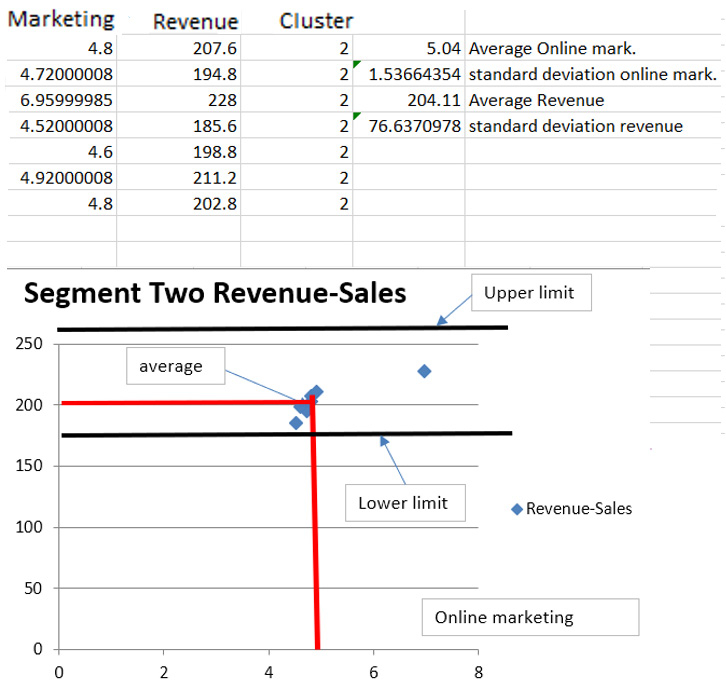Figure 1.9 – Segment two mean and standard deviation

As we are analyzing two variables, the centroid of the segment has two coordinates: the online marketing spend and the revenue.

The mean has the following coordinates:

• Online marketing: 5.04
• Revenue: 204.11

In Figure 1.9, the centroid is at these coordinates.

The standard deviation of online marketing is 1.53, and for revenue, it is 76.63.

The limits of the revenue are the black lines. They are 160 and 280. So, segment two is not compact because the majority of points are between 160 and 210 with an outlier close to 280.

When we analyze data with three variables, the mean and the standard deviation are represented by three coordinates. Figure 1.10 shows data with three variables and the segment that each of them belongs to: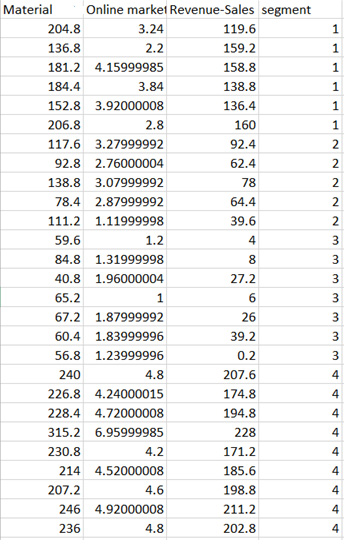Figure 1.10 – Segments with three variables

The mean and standard deviation have three coordinates. For example, for segment three, these are the coordinates: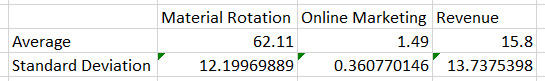Figure 1.11 – Mean and standard deviation coordinates with three variables

The standard deviation of revenue is large, 13.73. This means the points are widely scattered from the centroid, 15.8. This segment probably does not give accurate information because the points are not compact.

# Summary

In this chapter, we learned why it's important to find the optimal number of groups before we conduct K-means clustering. Once we have the groups, we analyze whether they are compliant with the best-case scenario for segments having a small standard deviation. Research outliers to find out whether their behavior could lead to further investigation, such as fraud detection.

We need a machine learning function such as K-means clustering to segment data because classifying by simple inspection using a 2D or 3D chart is not practical and is sometimes impossible. Segmentation with three or more variables is more complicated because it is not possible to plot them.

K-means clustering helps us to find the optimal number of segments or groups for our data. The best case is to have segments that are as compact as possible.

Each segment has a mean, or centroid, and its values are supposed to be as close as possible to the centroid. This means that the standard deviation of each segment must be as small as possible.

You need to pay attention to segments with large standard deviations because they could be outliers. This type of value in our dataset could mean a preview for future problems because they have a random and irregular behavior outside the rest of the data's normal execution.

In the next chapter, we will get an introduction to the linear regression supervised machine learning algorithm. Linear regression needs statistical tests for the data to measure its level of relationship and to check whether it is useful for the model. Otherwise, it is not worth building the model.

# Questions

Here are a few questions to assess your learning from this chapter:

1. Why is it necessary to know the optimal number of groups for the data before running the K-means classification algorithm?
2. Is it possible to use K-means clustering for data with four or more variables?
3. What are outliers, and how do we process them?

Here are the answers to the previous questions:

1. Having the optimal number of groups helps to get more compact groups and prevents us from having a large number of outliers.
2. Yes, it is possible. It is more difficult to visualize the potential groups with a chart, but we can use K-means clustering to get the optimal number of groups and then do the classification.
3. These are points that do not have the same behavior as the rest of the groups. It is necessary to do further research on them because it could lead to finding potential fraud or system performance degradation.

To further understand the concepts of this chapter, you can refer to the following sources:

• Eight databases supporting in-database machine learning:

https://www.infoworld.com/article/3607762/8-databases-supporting-in-database-machine-learning.html

• Creating a K-means model to cluster London bicycle hires dataset with Google BigQuery: